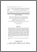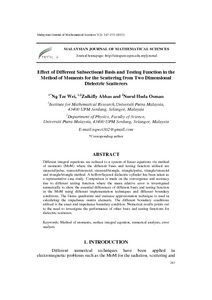# Effect of different subsectional basis and testing function in the method of moments for the scattering from two dimensional dielectric scatterers

## Citation

Ng, Tze Wei and Abbas, Zulkifly and Osman, Nurul Huda (2013) Effect of different subsectional basis and testing function in the method of moments for the scattering from two dimensional dielectric scatterers. Malaysian Journal of Mathematical Sciences, 7 (2). pp. 247-272. ISSN 1823-8343

## Abstract

Different integral equations are reduced to a system of linear equations via method of moments (MoM) where the different basis and testing function utilised are sinusoid/pulse, sinusoid/sinusoid, sinusoid/triangle, triangle/pulse, triangle/sinusoid and triangle/triangle method. A hollow/layered dielectric cylinder has been taken as a representative case study. Comparison is made on the convergence and accuracy due to different testing function where the mean relative error is investigated numerically to show the essential differences of different basis and testing function in the MoM using different implementation techniques and different boundary conditions. The Gauss quadrature and staircase approximation technique is used in calculating the impedance matrix elements. The different boundary conditions utilised is the exact and impedance boundary condition. Numerical results points out to the need to investigate the performance of other basis and testing functions for dielectric scatterers.Preview
PDF
30355.pdfView Item